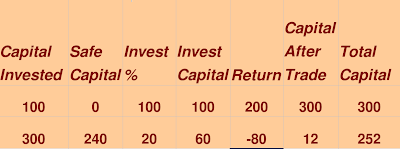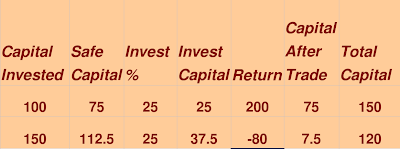# Game of Trading , Risk Management Part 1

POSTED BY ON January 9, 2009 COMMENTS (2)

Lets play a game, the name of the game is “Game of Trading”. I am stock market and you are investor. You have got 2 chances of investing you money, One time I will give you 200% return and other time I will give you -80%, or in reverse order, so it can either be

200%, -80%

OR

-80%, 200%

You have to decide in advance that how much percentage of your total capital you will invest each time (invest capital) and how much you will keep safe money (safe capital), you have to decide for both the times in the start only.

Lets analyse different cases.

#### Case A : You choose invest capital as 100% first time and 20% for next chance

Case A.1 : Return was -80% first time and 200% next time.Case A.2 : Return was 200% first time and -80% next time .

####Case B : You choose invest capital as 20% first time and 100% for next chance

Case B.1 : Return was -80% first time and 200% next time.Case B.2 : Return was 200% first time and -80% next time.You can see that at last A.1 = B.2 and A.2 = B.1 , so it means that order of your invest capital ratio does not affect your result , it both the cases it can either become 28 or 252 (depending on the return order) …

### What should you do?

100% and 20% choice will always loose in long run, if you play this game over and over again for long run, Understand that in this game, you can make it “high risk high return” Game or “Extremely no risk, low return game”, And your choice of your invest percentage will decide which game is it.

Characteristic of “High return High risk game” : Its possible to make great money in short term, but in long run you will loose.

Characteristic of “Low risk, low return game” : You will Not make great return in short term, but with compounding effect, you are bound to be a winner in long run.

Let see if we can choose a ratio (invest percentage) can give us some profit irrespective of the return order.

Lets choose 25% invest capital :

#### Case A.1 : Return was -80% first time and 200% next time.Case A.2 : Return was 200% first time and -80% next time.You can see that in any case your 100 becomes 120, which is 20% return.

What if you choose 80% invest capital : In that case at last you will have 93.6 (calculate yourself). So what should be the best percentage capital to deploy each time in this game.

#### I tried to make an Equation, with all variables

p = profit times (2 or 200/100)
l = loss times ( -.8 or -80%/100 )
C = Capital at the start
T = Trade factor (.25 means, 25% of the capital will be invested at any time)

We want to find optimum T, given any p and l (assuming that the trade will be done 2 times)

#### So, If you calculate the total capital after the 2 trades (do the math), you will get

Total capital = C (1 + pT) * (1 + lT)

So our original capital is getting multiplied by (1+pT)*(1+lT), and we have to maximize this number.

lets say I = (1+pT) * (1+lT)
I = 1 + plT^2 + pT + lT

If we do some differentiation here with respect to T (people who don’t know differentiation, just leave it), and put dI/dT = 0

2plT + p + l = 0
T = – (p + l) / 2pl

#### So the best valeuof T is -(p+l)/2pl ..

For our earlier example , p = 2 , l = -.8

we get – (2 – .8)/ (2 * 2 * -.8) = .375

Which means, 37.5% of capital will be invested everytime, and with that our capital will become 122.5 and that is the max you can make without risk.

What if return = 200% and -90% , in that case p = 2 , l = -.9 , so T = 2 – .9 / 2 * 2 * .9 = 1.1/3.6 = 11/36, means investing capital will be 30.555% always and that will give us max return.

What is the point i am trying to make?

In any given situation of making money, there may be a big risk of loosing it, we should always use these kind of tools and always be safe. Don’t try to be very bold in stock markets.

People who make killing in the start often get killed somewhere on the way and people who make respectable and sufficient money with satisfaction become winner over long term.

#### Summary

When you do Investment or do trading, you should never put all your capital into it, one bad trade or investment and you will be ruined forever, better to risk only that much capital which can not take out of of the game, but just hurts a bit.

Take small and risk-less profits if possible, Investing and trading is all game of probabilities. Use math’s and logic to take smart decisions like discussed in this article.

“There are old investors and there are bold investors, but not both”.

Check out this blog for Risk Management Part 2.## 2 replies on this article “Game of Trading , Risk Management Part 1”

1.kp says:

You above article don’t contain proper inputs. How many people get 200% gain in normal trading? for getting 200% I think u have to wait minimum 6-1 year and same goes for -80% return.

Generally If I do treading It will be in the range of 10-15% upside 2-5% down side.

If u use this params than your equation don’t make any sense.

How would u handle money allocation for short term treads(1-3months per tread).

1.Manish Chauhan says:

We are talking about 6 months to 1 yr time frame only .. we are talking about series of trades and the final profile and loss

This site uses Akismet to reduce spam. Learn how your comment data is processed.

#### FREE Financial Health Checkup

Take up a detailed 25 questions financial health checkup to find out how much you score out of 100?